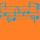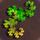## General Question# Can someone help me with this geometry problem?

Asked by dxs (14981) May 24th, 2013

First of all this has nothing to do with school or grades. I am making a building and am confused about how to find a certain angle.
I am looking for θ, and can’t seem to find it. θ is the angle from the dotted line (unknown length) to the line that is SQRT(9090)ft, and from the dotted line to the line on the bottom. Do I need to give you more information? Thanks for the help!
In case it is hard to read, here are the numbers on the diagram:
21’
93’
SQRT(9090)’
arctan(21’/93’)
67’6’’

Observing members: 0Composing members: 0Let’s designate as x the angle at the top of the right triangle and for convenience designate arctan(21/93) as k.

Using θ on the bottom and the exterior angle relationship gives:
θ = 90 + x

On the top we get
θ – k + x = 90

Substitute the first equation for θ in the second equation to get
90 + x – k + x = 90, which reduces to x = k/2
θ = 90 + x = 90 + k/2 = 90 + ½ arctan(21/93) = 96.36

LostInParadise (27296)“Great Answer” (5) Flag as…I don’t see how θ=90+x
θ – k + x = 90 would result in θ=0degrees.
: I get it now. x is at the top of the right triangle with 67’6” as a side. I was thinking that it was the compliment to k. Thank you so much for the help!

dxs (14981)“Great Answer” (2) Flag as…Although I am a bit late to this math party, I can confirm that @LostInParadise‘s answer is correct. I can, however, still contribute.

If you are designing a building, it might be useful to know the length of the dotted line.

180 – θ gives us one interior angle of the bottom-left right triangle. This vertex is opposite to the side that is 67.5 feet. We can use the law of sines to find L, the length of the dotted line.

67.5 / sin(180 – θ) = L / sin(90)
67.5 / sin(180 – 96.36218) = L / 1 (after some substitutions)
67.5 / sin(83.6378) = L
L is equal to around 67.9183 feet, or 67’11”.

PhiNotPi (12677)“Great Answer” (3) Flag as…@PhiNotPi Than you! I never usually think about using the law of sines when it comes to right triangles since I can also use a sine equation [sin(180—θ)=67.5/c].
I actually had to know the side between the right angle and 180—θ [tan(180—θ)=67.5/b] because I’m on Google Sketch-up, and I don’t know if there’s a protractor in the program anywhere, so I can’t measure out θ. I used that bottom side, which came out to 7’ 6”+5/16”, to draw out the hypotenuse. Being a pain that the program is (though it’s free so I can’t complain), you have to zoom in a lot to get the exact length you want, so it’d be hard to estimate the 67’ 11” onto the line on the bottom. You can’t just enter the length in or anything to my knowledge. It also helps to double-check your work on a “sketchy” program such as Sketch-up. haha…

dxs (14981)“Great Answer” (0) Flag as…There are a lot of packages for 2 dimensional drawings. Geogebra is free, but I prefer Geometer’s Sketchpad. It is not that expensive. You can specify and measure lengths. You can also measure angles and areas. There is a free trial version that lets you draw whatever you can do in 20 minutes. After that the drawing is lost, but it should give a good idea of how useful the program is.

LostInParadise (27296)“Great Answer” (1) Flag as…or